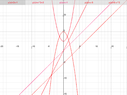# Circle a equation in a straight line

Abdullah Qureshi
how to circle a equation in a straight line
y= 2x-1
y = x^2+ 2
x –y = 1
x = y + 5
y = – x^2 + 5

pls help me

skeeter
You’ve posted the equations for three lines and two parabolas.
I have no idea what you are asking with regard to a circle.
Please translate the original problem to English and post it.Abdullah Qureshi
You’ve posted the equations for three lines and two parabolas.
I have no idea what you are asking with regard to a circle.
Please translate the original problem to English and post it.

https://www.physicsforums.com/attachments/11038
Can you without the graph

skeeter
Do what?

skeeter
Are you asking which of the five equations are lines?

HOI
how to circle a equation in a straight line
I don't understand what "to circle an equation in a straight line".
means.

y= 2x-1
This is the equation of a straight line.

y = x^2+ 2
This is the equation of a parabola.

x –y = 1
This is the equation of a straight line.

x = y + 5
This is the equation of a straight line.

y = – x^2 + 5
This is the equation of a parabola.

pls help me
There are NO circles.

Gold Member
MHB
It possibly means to encircle the equations forming straight lines.

Abdullah Qureshi
Do what?
Without making a graph. It can be use as simple way like trigonometry

Gold Member
MHB
Straight-line equations, or "linear" equations, graph as straight lines, and have simple variable expressions with no exponents on them. If you see an equation with only x and y, then you're dealing with a straight-line equation. As opposed to, say x² or √x .
The second and the last equations posted above have exponents, so they are not forming straight lines. The rest of them are. You can encircle the rest!

•Greg Bernhardt